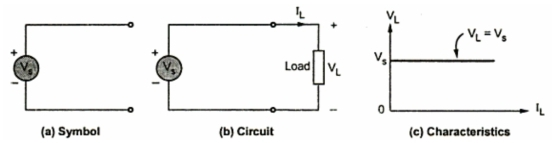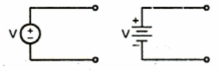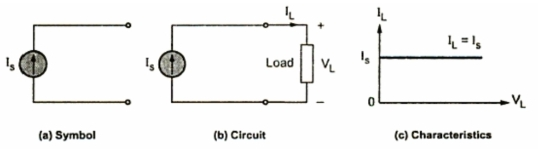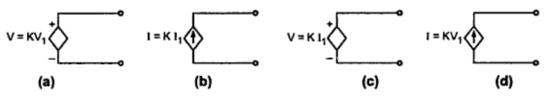### Energy Sources

There are basically tow types of energy sources ; voltage source and current source. These are classified as i) Ideal source and ii) Practical source.
Let us see the difference between ideal and practical sources.
1. Voltage source
Ideal voltage source is defined as the energy source which gives constant voltage across its terminals irrespective of the current drawn through its terminals. The symbols for ideal voltage source is shown in the Fig. 1(a). This is connected to the load as shown in Fig. 1(b). At any time the value of voltage at load terminals remains same. This is indicated by V-I characteristic in the Fig. 1(c).Fig 1  Ideal voltage source
Practical voltage source :
But practically, every voltage source has small internal resistance shown in series with voltage source and is represented by Rse as shown in the Fig. 2.Fig. 2  Practical voltage source
Because of the Rse, voltage across terminals decreases slightly with increase in current and it is given by expression,
VL = -(Rse ) IL + V = VS - IL Rse
Key point : For ideal voltage source, Rse = 0
Voltage source are further classified as follow,
i) Time invariant sources :
The sources in which voltage is not varying with time are known as time invariant voltage sources or D.C. sources. These are denoted by capital letters. Such a source is represented in the Fig. 3(a).Fig.  3 (a) D.C source
ii) Time variant sources :
The sources in which voltage is varying with time are known as time variant voltage sources or A.C. sources. These are denoted by small letters this is shown in the Fig. 3(b).Fig. 3 (b)  A.C. source

2. Current sources
Ideal current source is the source which gives constant current at its terminal irrespective of the voltage appearing across its terminals. The symbol for ideal current source is shown in the Fig. 4(a). This is connected to the load as shown in the Fig. 4(b). At any time, the value of the current flowing through load IL is same i.e. is is irrespective of voltage appearing across its terminals.This is explained by V-I characteristics shown in the Fig. 4(c).Fig. 4  Ideal current sorce
But practically, every current source has high internal resistance, shown in parallel with current source and it is represented by Rsh . This is shown in the Fig. 5.Fig.  5    Practical current source

Because of of Rsh , current through its terminals decrease slightly with increase in voltage at its terminals.
Key point : For ideal current source, Rsh = ∞
Similar to voltage sources, current sources are classified as follow :
i) Time invariant sources :
The sources in which current is not varying with time are known as i) Time invariant sources or D.C. sources. These are denoted by capital letters. Such a current source is represented in the Fig. 6(a).Fig. 6 (a) D.C. source
i) Time variant sources :
The sources in which current is varying with time are known as i) Time variant sources or A.C. sources. These are denoted by small letters.Such a source is represented in the Fig. 6(b).Fig. 6 (b) A.C source

The sources which are discussed above are independent sources because because these sources does not depend on other voltages or currents in the network for their value. These are represented by a circle with a polarity of voltage or direction of current indicated inside.

3. Dependent sources
Dependent sources are those whose value of source depends on voltage or current in the circuit. Such sources are indicated by diamond as shown in the Fig. 7 and further, classified as,
i) Voltage Dependent Voltage Source : It produce a voltage as a function of voltages elsewhere in the given circuit. This is called VDVS. It is shown in the Fig. 7(a).
ii) Current Dependent Current Source : It produces a current as a function of currents elsewhere in the given circuit. This is called CDCS. It is shown in the Fig. 7(b).
iii) Current Dependent Voltage Source : It produces a voltage as a function of current elsewhere in the given circuit. This is called CDVS. It is shown in the Fig. 7(c).
iv) Voltage Dependent Current Source : It produces s current as a function of voltage elsewhere in the given circuit. This is called VDCS. It is shown in the Fig. 7(d).Fig.  7

K is constant and V1 and I1 are the voltage and current respectively, present elsewhere in the given circuit. The dependent sources are also known as controlled sources.

#### 1 comment:

1.Amazingly informative and helpful post.Everything is good enough and website is very well designed.I am very glad that I have come across to your blog because you have shared a one of a kind blog which has all the things in a very pleasant manner.Basically I'm a writer I would like to share my website here thesis writing service and sometimes I wait for your post to get more this type of blogs.A good blog always comes-up with new and exciting information and while reading I have feel that this blog is really have all those quality that qualify a blog to be a good one. What a great post, love this education related post! Great blog you have Thanks for sharing.